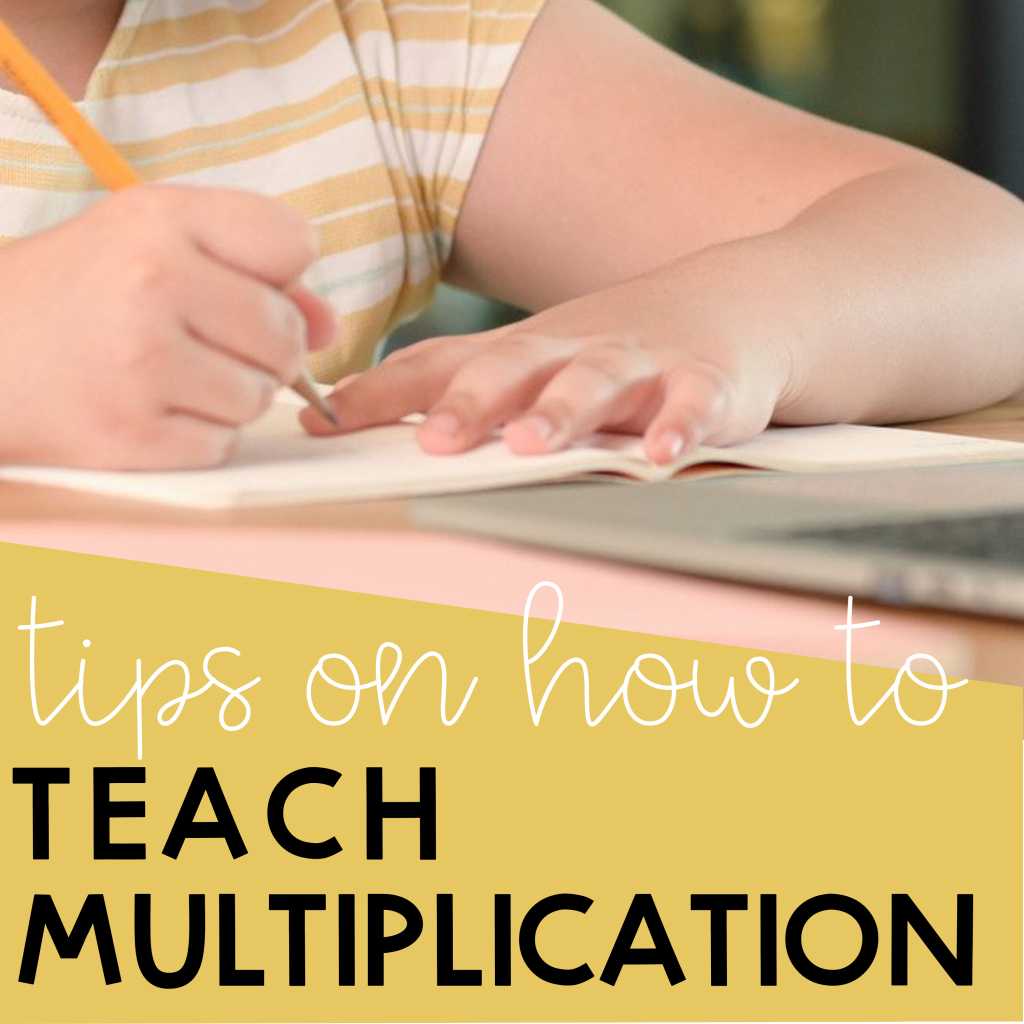# Teach Multiplication Effectively

Are your students struggling to learn their multiplication facts? Perhaps you need to take a step back and put down the multiplication table. Children learn better when they are engaged. Below are some tips you can use to teach multiplication effectively.

## Tip #1: Make multiplication meaningful

Oftentimes you see students struggling to learn their multiplication table because they can’t relate to it. To teach multiplication in a more meaningful way, you have to engage your students. One way you can do this is by posing problems that they can relate to. Be intentional with the word problems that you use and let them learn the language of multiplication by using phrases such as ‘groups of,’ ‘times as many as,’ ‘jumps of,’ or  ‘each.’ Prompt them to notice the words used in the word problems so that they can come up with a connection between the words and multiplication.

Here are some examples of word problems that you can use to teach multiplication.

• I have three students. I give them four pencils each. How many pencils did I give in all?
• You are baking cookies. You place them in three rows and there are four cookies in each row. How many cookies did you bake in all?
• A snail moves without stopping for four minutes. He moves three inches for each minute. How far does the snail move?

## Tip #2: Make multiplication visual

Another way you can engage your students is to use visuals when you are teaching multiplication. Drawing equal groups, making arrays, or using a number line are helpful tools when visualizing multiplication. These will give a clearer picture of what multiplication does. Let’s look at each of them.

### Use equal groups to teach multiplication

Now, let’s look at equal groups. When teaching multiplication, equal groups is a useful visual. So, what are equal groups? It is an equal number of groups so that each group has the same number of items as the other groups. Now let’s look at the first word problem from tip #1.

Since it was mentioned that the teacher gave the same number of pencils to each of the students, the problem asks for equal groups. So you’ll need 3 groups of 4. One way you can show 3 groups of 4 is by drawing big circles to show the number of groups (the number of students) and drawing smaller circles inside each group to show how many (the number of pencils).  This way, you can clearly show the number of pencils given by counting the total number of smaller circles.

### Make arrays to teach multiplication

Second, let’s look at making arrays. Arrays are an arrangement of objects or pictures in rows and columns. Let’s look at the second problem and model it using arrays.

To model this problem, start by making 3 rows and making sure that there are 4 objects in each row. You can use any object such as circles or squares to help visualize multiplication. Notice how the word problem relates to the model. This will help your students to be more visual when they are multiplying.

### Use number lines to teach multiplication

Another useful tool when teaching multiplication is to use number lines. Number lines are great visual tools for multiplication to show ‘skips’ or ‘jumps.’

The third problem will help visualize this. The number of jumps that the rabbit makes is the number of groups and the length of each jump would tell you how much you need to make for each jump. By using a number line and drawing 3 jumps of 4, students will be able to visualize multiplication.

These are just some models that your students can use to show multiplication. Other than drawing, you can also use physical manipulatives to make the concept of multiplication even more graspable. Let them explore the many different ways to show multiplication.

## Tip #3: Look for Patterns

Patterns are everywhere, especially in Math. To be able to teach multiplication effectively, help your students notice and look for patterns. One effective thing to do is to show your students a table and skip count by a number to show our multiplication facts. After skip counting, prompt them to notice patterns other than skip counting.

One example is the multiplication facts of 4 as shown below.

Prompt your students to notice the ones digit. In multiplication tables of 4, the ones digit alternate 4, 8, 2, 6, or 0. Another pattern is that they are all even numbers. This will help with their fluency later on when they are multiplying multi-digit numbers. It would help them see if their answers are reasonable.

For you to teach multiplication effectively, try these tips!

Are you looking for more multiplication resources? Check out these products on Teachers Pay Teachers!